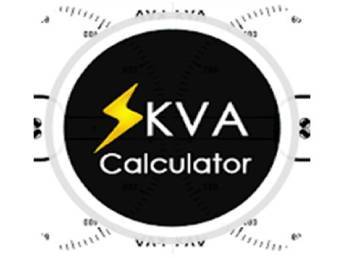# What is kVA and how is it calculated?Generator

kVA Power Rating

Power ratings are expressed in different forms such as WATTS, AMPERES or AMPS, VOLTS, and also in KVA, But what exacty is kVA…

Outside the diesel generator industry, the term Kilo-volt-amperes (kVA) is not well known. A Kilowatt (kW) is much more commen a term and is how electrical items in your home are rated, you may even notice it quantified on your electricity bill so it is much more relatable but what is kVA?

kVA Actual Power

Therefore we can refer to kW as actual power, it is the amount of power that is converted into an output.

kVA Aparent Power

On the other hand kVA is a measure of apparent power: it describes the total amount of power being used by a system, for example in a 100% efficient system kW would equal kVA exactly. However in reality electrical systems are not 100% efficient and so not all of the systems apparent power is being used for useful work output. Fundemantally one kVA is equal to 1,000 volt amps. Whereas a volt is for measurement of electrical pressure an amp is a way of electrical current mesurement. A term called apparent power (the absolute value of complex power, S) is equal to the product of the volts and amps.

kVA Power Factor

Diesel Generators have a power factor of 0.8. When you know this, it is easy to convert kVA to kW because you know the efficiency level of the electrical system in question. Electrical efficiency is usually expressed as a power factor in between 0 & 1, therefore the closer the power factor is to 1, then the more efficiently the kVA is being converted into actual killerwatts.

### THE KVA INTO KW FORMULA:

Apparent power (kVA) x power factor (pf) = actual power (kW)

e.g. 100 kVA x 0.8 = 80 kW

The formula for converting kW into kVA is:

Actual power (kW) / power factor (pf) = apparent power (kVA)

1 ton = 200 BTU/minute

1 ton = 12,000 BTU/hour

1 ton = 3.517 kilowatts

Convert kVA into KW kVA to KW kVA to KW conversion.